## Monday, August 31, 2020

### Darlington amp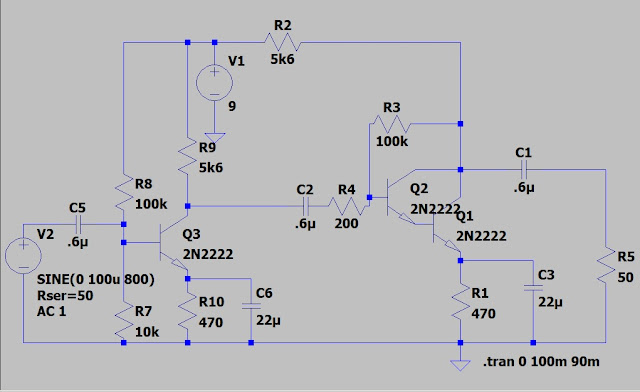Someone asked about a circuit such as this on a radio forum. I responded "try it you may like it". The goon squad informed us it was a stupid idea and would eat a battery in no time.So, i had to try it. My circuit draws less than 3ma. I put a coil and diode on the input and could hear our local station .
In the sim I used a pair of 2N2222's on board I used a KSP13.

## Friday, August 28, 2020

### Using an ohmmeter and resistor to ID a transistor pins

If you need to ID a transistor's leads you can use an ohmmeter to find the lead that shows continuity to the other two leads. Then what?For sim to work I must have a ground reference so I grounded one side of the battery. The battery and resistor represent an ohmmeter. This meter would have a 1ma movement and is reading full scale or zero ohms.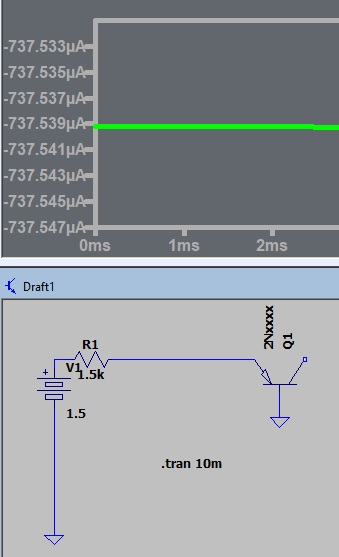When connected across the emmiter and base I read the junction forward bias resistance.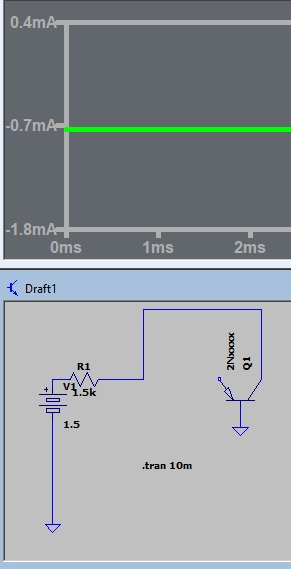I also get a forward junction reading between the base and collector.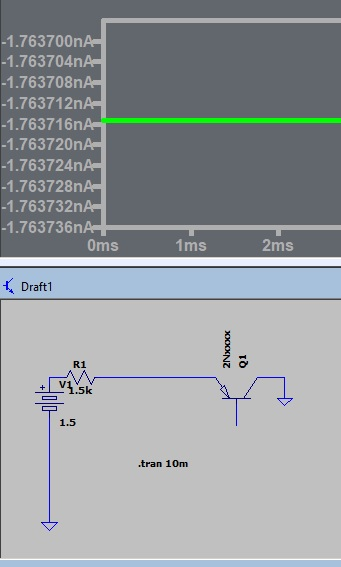The emmitter collector reading is very high.connecting the resistor between the base and emitter and base produces no current flow or a high resistance.When the resistor is placed between the base and collector we see transistor action. The high current represents a low resistance reading on the meter. We have proved the transistor pinout and that it will produce a gain.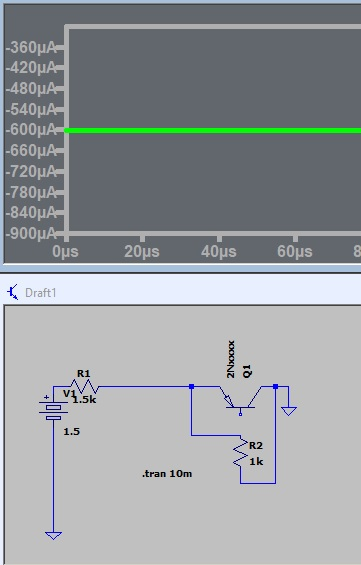Placing the resistor from emitter to collector will produce a reading representing the 1k resistor, in this case .6ma. the previous .76ma represented a lower reading. So to repeat the meter reads lower than the 1k resistor proves the transistor connection and function.
In summary connect one metter lead to a pin and check between the other 2. Find the pin that is common when reading between the other 2. This is the base. Conect the meter across the other 2 pins and use the resistor to locate the collector. Simple?

## Wednesday, August 26, 2020

### Consider the output as a capacitive divider

A short time ago DIT posted a cap divider. Let's take a short review and then consider the amp output with respect to a cap divider.

1 the capacitor will establish a max current

2 the load will pass that current as long as we maintain a ratio of less than 10 to 1

3 when the load equals the source we get max power transfer but only half the current.

Examine the screen shots and you will see as I step the load resistor the current remains constant as long as I keep the collector resistor 10 times the load.

You should also see the current threw the emitter bypass is equal to the load current.

When I set the collector equal to the load the current is half.

The directive above the load resistor tells the sim to step the value of the resistor. As it is stepped the current is traced on the display.

NOTE: I made the 3 transistor version and it was to sensitive. I removed the first stage and it is giving a 37db gain.

## Tuesday, August 25, 2020

### mind experiment in amp design part 4

Why would I use the feedback amp design?
It is STABLE. (I emphasize this because someone has stated the opposite)
The input and output Z can be established by selecting the bias resistors.
Why is Z matching important? For an AF amp not so much but signal phasing will effect stability and I could build an oscillator if I don't pay attention to the loading /phasing. The collector signal being 180 degrees out of phase with the input produces degeneration and stability.

It would be good to examine the TD and reflection of Transmission lines. Then consider any conductor carrying a signal is a transmission line. At audio and low RF frequencies we can ignore these principles and get by but as frequency increases we much pay attention to them.

## Wednesday, August 19, 2020

### Mind experiment in amp design part 3Using the opamp design as our basis we would expect a gain of 100 with the 1k to 100k feedback bias network and we expect 50mv out with the load matching the source Z. Q1 load of 4k7 matching R4 delivers that 50mv. This is a very useful circuit and fairly easy to design once you learn the design considerations.

As stated in the opamp design I would expect the output to be 100 with the load set at 10 times the R1. What happened? Remember the opamp is capable of a gain of 100,000. The transistor is limited to a much lower gain. R3 is setting the base current which in turn sets the gain so we need a little more consideration in the component values.

I reduced R2 and increased R4 and the gain goes to 200.
Matching the load brings the gain back to 120.
Increasing R2 gives the desired gain of 100 with the load matched.
Here we see the frequency response for the circuit. Adjusting the capacitors will give the response we need.
What can you deduce from this series?
A low Z circuit is high current and a high Z circuit is low current. Can we design the circuit to be voltage or power gain? For voltage gain would you want high or low Z?

## Monday, August 17, 2020

### Mind experiment in amp design part 2

The last post explained how to set the gain of an opamp with the feedback resistors. Now that we know how to set the gain we will look at the effect of loading on the output. We need to look at the generator Z and the load Z The old aying is for max power transfer we need Zgen = Zload but it is not always that simple. consider a power plant generator producing 100 megawatts with the Zgen - Zload. It would send 50 megawatts to the city and drop 50 megawatts internally. Obviously we do not want that! The other old saying is to make Zgen 1/10th Zload. In my previous example we would still have 10 megawatts dissipated in the generator. In some cases a 1000 to 1 ratio is still potentially a problem. It is fairly simple make a single stage amp. The beginner will often find it more difficult to build a 2 stage amp because of the Z matching.

Examine the circuit to determine the gain. With 10k and 1000k resistors the gain should be 1000k/10k = 100. The gain of 14 is far from it! Remember the generator has internal drops. In this case it is dropping 86% of the signal. Not good at all.

By increasing the load Z we now have 100mv output which is our calculated value.

I "Z matched" the amp with a 220 ohm load and get maximum power transfer and 50 mv. If I had a high Z speaker with a Z of 220 ohm this would be good. For a voltage amp not so good. So the amp can produce power or voltage gain. The Z matching would be adjusted accordingly.

## Sunday, August 16, 2020

### Mind Experiment in amp design.

I will try a little experiment with design considerations and then run a sim to determine if the theory is sound.

NOTE: This is my own observations. Not accepted ideas.

We assume the amp is capable of infinite gain, the + input is non inverting , the - input is inverting and zero potential between - and + produces zero output. 10m input with the circuit as drawn should produce 100mv out. The circuit is not infinite gain so the output is a little less than 100mv. (the actual gain for an opamp maybe 100k.)

With the feedback resistors set to 1k and 100k what is the gain?

Resetting the feedback resistors to 1k and 1000k should give what output?

Changing the input to 10m should produce what output with the 1k and 1000k feedback circuit?

What limits the output?

I bumped the input a little more to emphasize the limit at rail voltage.

Next we look at the output circuit. The unloaded amp operates according to the basic theory of feedback resistor ratios control the operation. What will happen when we load the circuit?

## Monday, August 3, 2020

### LDO DC receiver cross feed vs shielding part 2 add DBM

Looking at the LO , RF and OUTPUT. You can see the inputs mix and produce a difference (lower frequency) output.

I added an inductor in each input leg with no ill effect.

When I "LINK" the inductors the output is lost.
So the point is this.
The LO is producing millvolts of signal and the RF is microvolts so we must prevent the LO feeding the antenna connection.
What can we do?

### LDO DC receiver cross feed vs shielding part 1 the oscillator

The tunnel diode oscillator would be ideal for our DC receiver but they are not readily available so I will be looking at the LDO.

Using 2 JFETs we have a LDO.